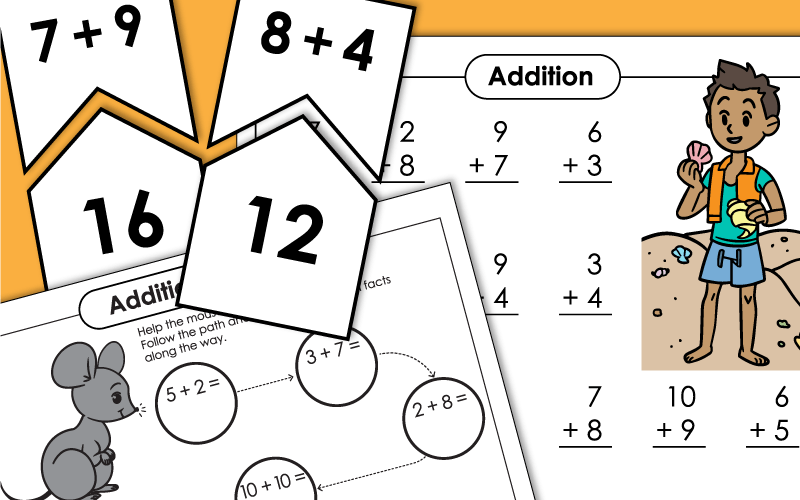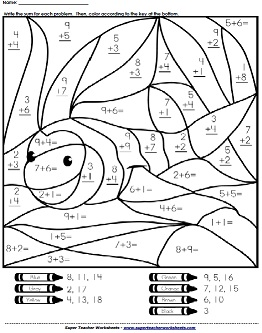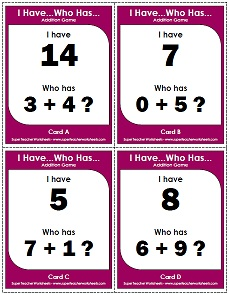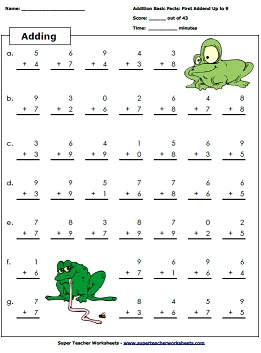# Basic Addition (Sums to 20)

Here is a collection of basic addition worksheets, activities, and games. These worksheets include single-digit addition facts (with addends 0-10). Students can practice memorizing the facts to build speed and accuracy.Add together the red and white counters to find the sum.
Count the dots on each domino and add to find the sum.
Help the mouse find the cheese. Along the way, answer the addition facts with sums to 20.
Add the counters shown on the ten frames and write the sum.
Cut out the boxes on the bottom of the worksheet and use a glue stick adhere the sum to the correct math fact.
Cut out the pieces to these 6
star-shaped puzzles. Then place the problems with the same sum to make stars.
A set of 30 boards for addition bingo, and calling cards.
Read each word problem (addition story). Draw a picture to solve. Write a number sentence (equation) for each.
Memory match card game.  Flip cards to match each addition fact with the correct domino.
Scoot! is a fun game that the whole class can play together. Students move to each desk in the classroom, solving basic addition problems. Game includes 30 task cards, teacher instructions, and a student answer worksheet.
Roll two dice. Write the numbers and add them together to find the sum. This version is for one player.
Players take turns rolling dice and adding the numbers to find the sums. In each round, the player with the greatest sum wins.
In this game, kids take turns rolling a die. The number rolled corresponds with a column of math facts on the game board. They read the column of addition facts (with answers) as quickly as possible.
Using this printable activity, students can find their matching puzzle piece by solving basic addition problems.
Write the domino addends, then count the dots to find the correct sum.
Write the answers to the addition facts on the sections of the caterpillar.
Tell which addition problems are shown by each number line.
Students must determine which facts are correct, and which ones are incorrect. They write the sums for each incorrect fact.
This fun chain reaction game that teaches basic addition facts.
Try to find and circle all of the addition facts hidden in this number hunt puzzle.  It's kind of like a word search, except students search for addition facts.
Logical thinking:  Write the missing numbers to make each scale balance.

Write the sum for each addition problem, then color the parrot according to the key.
Depending on which worksheet from this file you give your students, they will color either a dog or a cat.
Color a king and queen's castle.  Solve the basic adding facts and color the picture.
Complete the addition problems and color the page according to the key. The two worksheets have the same pattern, but when colored in, each will reveal a different picture.
Which worksheet they complete will determine whether your students color in an apple or a banana.
Solve the simple one-digit addition problems, and color the hot air balloon picture according to the code.
Solve the addition problems, the color-by-number according to the key.  Picture makes a colorful rooster.
Answer the addition facts and color the rocket picture according to the code at the bottom.
Will this mystery picture be a boat or a plane? It depends which worksheet you give your students!
After answering the addition facts, color the sailboat according to the key.
Solve the basic addition problems. Each problem has addends up to 9 and sums up to 18. Then color the summer scene according to the key.
Find the sum for each problem, then color the mystery picture according to the code.
The final picture on this worksheet shows an orange-bellied mama bird, sitting on eggs in her nest.
Solve the facts and color this adorable brown dog with black spots.
Each problem has a sum of 16 or less. After writing the answers, color the dragon picture according to the key.
Solve the problems to reveal fruits and vegetables: a red apple, a yellow banana, green guava fruit, and an orange carrot.
Solve the addition problems and color the worksheet based on the key. Depending on which you complete, you will create either a police car or a fire truck.
Solve the addition problems and color the sums to reveal an owl. (Note: This picture requires students to have 3 shades of brown crayons.)
On this worksheet, a ladybug is revealed when students correctly find the sums to basic addition facts.
Color the blue whale mystery picture after solving the basic addition facts.
Practice basic addition and create a fun coloring picture with this Easter-themed mystery picture.

Make your own basic addition worksheets. You choose the range of the addends. Generate worksheets with 25 or 50 problems.

## Math Fact Practice

This worksheet has 20 addition problems for students to solve. Addends are 10 or less and sums are up to 20.
Print out this worksheet to practice basic addition problems with sums up to 20.
Write the answers to twenty addition facts and color the pictures, if you wish. Addends are 10 or less. Sums are up to 20. (examples: 6+10=16 and 9+9=18) This worksheet features illustrations of kids holding seashells.
Color and solve these 20 math problems. Addends are 10 or less. Sums are 20 or less. (examples: 6+9=15 and 7+7=14)
Illustrations of kids on a bike and a skateboard are featured on this worksheet.
Each of these facts has addends up to 10 and sums of 20 or less. Illustrations show an kids outside in the rain.
This printable addition worksheet has twenty addition problems to be solved. The addition facts on this worksheet are listed horizontally with addends up to 10 and sums up to 20.

Practice worksheet with basic addition facts.  The first addend is between 0 and 2.  The second addend is between 0 and 9.  Includes pictures of cute monsters.
Practice sheet with forty-three basic addition facts.  The first addend ranges from 0 to 3.  Includes pictures of roller coasters.
Adding - Basic facts practice drill worksheet.  The first addend ranges from 0 to 4.  Includes pictures of horses.
Adding practice sheet.  The first addend ranges from 0 to 5.  Playful monkey pictures decorate the worksheet.
Another basic facts drill.  The first addend is between 0 and 6.  This worksheet has race car graphics.
More basic addition fact practice.  The first addend is between 0 and 7.  Kitty cat pictures.
Basic addition facts.  The first addend for each problem is between 0 and 8.  Cute wizard pictures.
Basic addition worksheet.  First addend is between 0 and 9.  Includes cartoon frog pictures.

Try to write the answers to all 50 Basic Addition Facts (0 -9) as quickly as possible.
On this printable timed test, students will have 50 more basic addition problems to solve.

## Flashcards

This is a set of flashcards up to 10 + 10. This set has 9 cards per page.
Complete set of printable addition flash cards up to 9 + 9. This set has four large cards per page.
Here's a complete set of triangular fact family flashcards to teach subtraction and addition facts.

## Classroom Display

This file has 100 basic fact pennants you can hang in your classroom. If you'd like a black-and-white version that children can color, click on the "ALT" button below.

Find sums for all basic facts on the addition table.
Here's another version of the addition table. This one has a pink bear graphic at the top.
This version of the adding table has a blue bear at the top of the page.
Complete the mixed-up addition table by writing sums in the empty squares.
This page has four mini charts that you can cut apart and give to students.
Here's a more basic addition table, with facts up to 5.

Add two, three, and four digit numbers, Adding money amounts, and more.

Add columns of one, two, three, and four-digit numbers.

These number family and number bond worksheets will teach students about the relationships between addition and subtraction.

Sum Game

Print the game boards and color pairs of adjacent numbers with a given sum.

These worksheets, flashcards, and number lines have a mix of basic addition and basic subtraction problems.My Account
Site Information